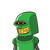# Find the perimeter of the triangle whose vertices are (-2, 1), (4, 6) and (6, -3).​

Find the perimeter of the triangle whose vertices are
(-2, 1), (4, 6) and (6, -3).​

### 1 thought on “Find the perimeter of the triangle whose vertices are<br /> (-2, 1), (4, 6) and (6, -3).​”

1.use distance formula let points be A(-2,1),B(4,6),C(6,-3)

Step-by-step explanation:

AB= √(-2-4)^2+(1-6)^2

=√36+25

= √61

similarly BC=√85

CA= √80

find sum of all sides u will get ur answer